In mathematics, we calculate the volume and surface area of a sphere. We need to learn the formulas, and we can understand how to calculate the volume and surface area of a sphere by using the formulas.

The formula that produces the volume and surface area of a sphere is a bit complicated. Also, to explain why the formula is true, we have to learn high school math integration. The content is therefore difficult, and we will not explain in detail why the formula is correct.

Instead, we will explain how to use the formula to calculate the volume and surface area of a sphere.

In math calculation problems, spheres are infrequently asked. Nevertheless, it is a field in which we have to remember the formulas. So, try to understand how to calculate it.

## How to Get the Volume of the Sphere Using Formula

In mathematics, we may be asked to calculate the volume of a sphere. Spheres are familiar to us, and many people use them in sports. For example, we use a ball in tennis, soccer, basketball, and volleyball.

The following three-dimension objects are called spheres in mathematics.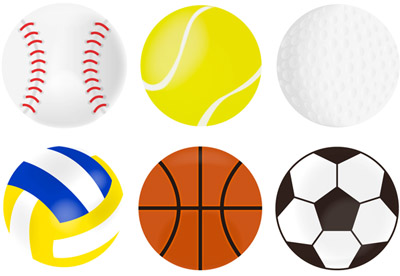To calculate the volume of spheres, we use the formula. If you do not remember the formula, you will not be able to find the volume of the spheres. Here is the formula for finding the volume of a sphere.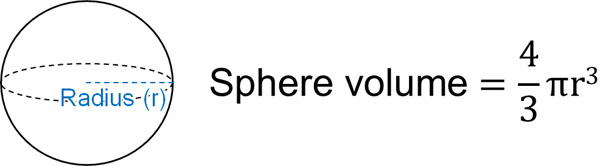If we substitute a number into this formula, we can get the volume of the sphere.

### The Relationship Between the Cylinder and the Sphere Volume

The sphere has a unique property. If the sphere is exactly inside a cylinder, the volume of the sphere is $\displaystyle\frac{2}{3}$ of a cylinder.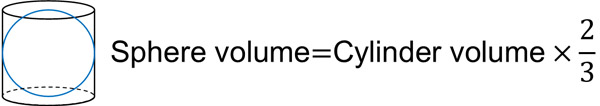By using this property, we can get the volume of the sphere.

Let the radius of the sphere be r. In this case, the height of the cylinder is 2r. The volume of the cylinder can be calculated by multiplying the base area and height. Therefore, the volume of the sphere is as follows.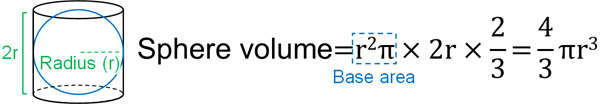The volume of a sphere can only be calculated if we remember the formula. However, if we understand that multiplying the cylinder volume by 2/3 to get the sphere volume, we can derive the formula.

### The Volumes of Cones, Spheres, and Cylinders are Interrelated

By the way, cones, spheres, and cylinders are related to each other. The cone volume is one-third of the cylinder. The sphere volume, on the other hand, is 2/3 of the cylinder.

In other words, the volume ratio of each is as follows.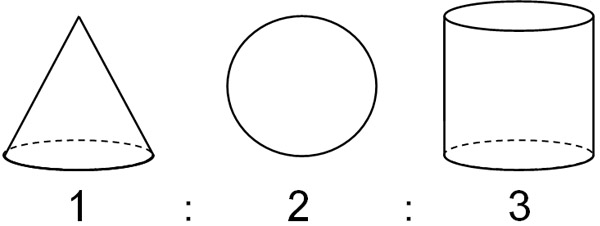When doing math calculations, you must remember the formula for a cone (or a pyramid). Problems that ask for the volume of a pyramid or cone are frequently asked. On the other hand, the formula to find out the volume of a sphere is not as important as a cone.

Even if we forget it, we can still make the formula for the sphere if we remember the following relationship.

• Cone : Sphere : Cylinder = 1 : 2 : 3

Multiply the cylinder by 1/3 to get the volume of a cone. In this case, double the volume of the cone is the sphere. Also, if we multiply the volume of the cylinder by 2/3, we get the volume of the sphere.

## How to Find the Surface Area of a Sphere Using a Formula

Just as there is a formula to produce the volume of a sphere, there is also a formula to produce the surface area of a sphere. As with the formula for volume, we must remember the formula to calculate the surface area of a sphere.

The formula for producing the surface area of a sphere is as follows.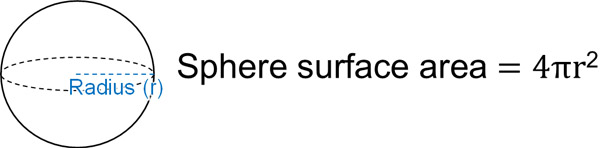If we want to calculate the surface area of the sphere, let’s substitute a number into this formula.

### The Relationship Between Side Area of a Cylinder and Surface Area of a Sphere

But it is hard to remember the formula for the surface area of a sphere. Like the sphere volume, it is used less often. How can we understand this formula?

The surface area of a sphere also has a unique characteristic. It has the property that if a sphere fits perfectly inside a cylinder, the surface area of the sphere is equal to the side area of the cylinder.

If the radius is r, the horizontal length of the side area of the cylinder is $2πr$. In other words, the diameter of the circle is 2r, and we can multiply it by pi to get the circumference (the horizontal length of the rectangle). The height of the cylinder, on the other hand, is 2r.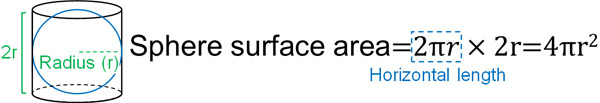If we forget the formula for the surface area of a sphere, we can still derive the formula if we know that it is equal to the side area of the cylinder.

## Exercise: Volume and Surface Area of the Sphere

Q1: Calculate the volume and surface area of the following hemisphere. Let the pi be $π$.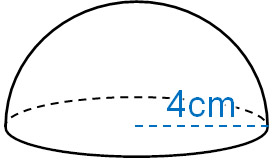-Volume of the hemisphere

Since it is a hemisphere, its volume is half of a sphere. If we apply the formula to get the volume of the sphere, it is $24π$ cm3 as follows.

• $\displaystyle\frac{3}{4}π×4^3×\displaystyle\frac{1}{2}=24π$

-Surface area of the hemisphere

The surface area is half of a sphere. Substituting the numbers into the formula for calculating surface area, we can calculate the following.

• $4π×4^2×\displaystyle\frac{1}{2}=32π$

We must also add the area of the cross-section of the hemisphere. Substituting into the formula for the area of a circle, we can calculate the following.

• $4×4×π=16π$

The total area is $48π$ cm2 as follows.

• $32π+16π=48π$

## Solving the Sphere Problem Using Formulas

One of the areas studied in mathematics is the volume and surface area of a sphere. Although this is not a frequently asked math problem, we need to remember the formulas to solve the problems.

Since we don’t use the sphere formula many times in math calculation problems, it is easy to forget the formula. So, try to understand how to come up with the sphere formula. Even if we forget, we can create the sphere formula from the formula that produces the volume or the side area of a cylinder.

• A sphere volume: 2/3 of the cylinder volume
• The surface area of a sphere: equal to the side area of the cylinder

If you remember this property, you can make a formula for the sphere at any time. Then use the formula to get the volume and surface area of the sphere.